Please wait a minute...
 Chin. Phys. B, 2020, Vol. 29(10): 104601    DOI: 10.1088/1674-1056/ab9ddf
 ELECTROMAGNETISM, OPTICS, ACOUSTICS, HEAT TRANSFER, CLASSICAL MECHANICS, AND FLUID DYNAMICS Prev   Next

# Anti-plane problem of nano-cracks emanating from a regular hexagonal nano-hole in one-dimensional hexagonal piezoelectric quasicrystals

Dongsheng Yang(杨东升) and Guanting Liu(刘官厅)†
1 College of Mathematics Science, Inner Mongolia Normal University, Hohhot 010022, China
Abstract

By constructing a new conformal mapping function, we study the surface effects on six edge nano-cracks emanating from a regular hexagonal nano-hole in one-dimensional (1D) hexagonal piezoelectric quasicrystals under anti-plane shear. Based on the Gurtin–Murdoch surface/interface model and complex potential theory, the exact solutions of phonon field, phason field and electric field are obtained. The analytical solutions of the stress intensity factor of the phonon field, the stress intensity factor of the phason field, the electric displacement intensity factor and the energy release rate are given. The interaction effects of the nano-cracks and nano-hole on the stress intensity factor of the phonon field, the stress intensity factor of the phason field and the electric displacement intensity factor are discussed in numerical examples. It can be seen that the surface effect leads to the coupling of phonon field, phason field and electric field. With the decrease of cavity size, the influence of surface effect is more obvious.

Keywords:  one-dimensional quasicrystals      piezoelectricity      surface effect; energy release rate
Received:  19 March 2020      Revised:  18 June 2020      Published:  05 October 2020
 PACS: 46.05.+b (General theory of continuum mechanics of solids) 46.25.-y (Static elasticity) 46.50.+a (Fracture mechanics, fatigue and cracks)
Corresponding Authors:  Corresponding author. E-mail: guantingliu@imnu.edu.cn
About author:
†Corresponding author. E-mail: guantingliu@imnu.edu.cn
* Project supported by the National Key R&D Program of China (Grant No. 2017YFC1405605), the Innovation Youth Fund of the Ocean Telemetry Technology Innovation Center of the Ministry of Natural Resources, China (Grant No. 21k20190088), the Natural Science Foundation of Inner Mongolia, China (Grant No. 2018MS01005), and the Graduate Students’ Scientific Research Innovation Program of Inner Mongolia Normal University (Grant No. CXJJS19098).

#### Cite this article:

Dongsheng Yang(杨东升) and Guanting Liu(刘官厅)† Anti-plane problem of nano-cracks emanating from a regular hexagonal nano-hole in one-dimensional hexagonal piezoelectric quasicrystals 2020 Chin. Phys. B 29 104601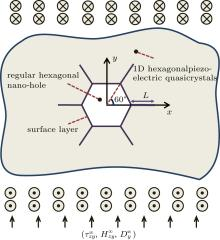Fig. 1.  Regular hexagonal nano-hole with six nano-cracks in 1D hexagonal piezoelectric QCs.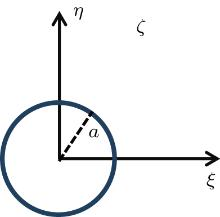Fig. 2.  Conformal mapping (ζ = ξ + iη).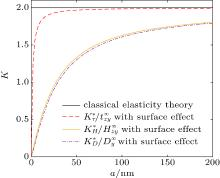Fig. 3.  With surface effect: when only the phonon field stress is applied, variations of ${K}_{\tau }^{* }/{\tau }_{zy}^{\infty }$ with a; when only the phason field stress is applied, variations of ${K}_{H}^{* }/{H}_{zy}^{\infty }$ with a; when only the electric field stress is applied, variations of ${K}_{D}^{* }/{D}_{y}^{\infty }$ with a. Without surface effect: classical elasticity theory.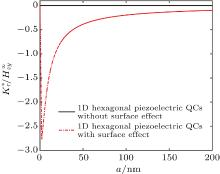Fig. 4.  Variations of ${K}_{\tau }^{* }/{H}_{zy}^{\infty }$ with a.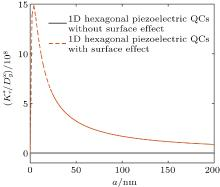Fig. 5.  Variations of ${K}_{\tau }^{* }/{D}_{y}^{\infty }$ with a.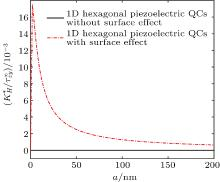Fig. 6.  Variations of ${K}_{H}^{* }/{\tau }_{zy}^{\infty }$ with a.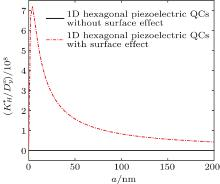Fig. 7.  Variations of ${K}_{H}^{* }/{D}_{y}^{\infty }$ with a.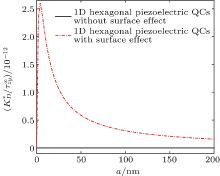Fig. 8.  Variations of ${K}_{D}^{* }/{\tau }_{zy}^{\infty }$ with a.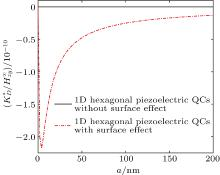Fig. 9.  Variations of ${K}_{D}^{* }/{H}_{zy}^{\infty }$ with a.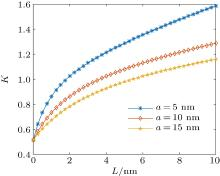Fig. 10.  Variations of K with L for some given a.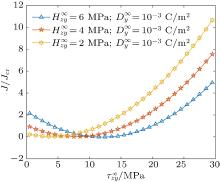Fig. 11.  Variations of J/Jcr with ${\tau }_{zy}^{\infty }$ for some given ${H}_{zy}^{\infty }$ .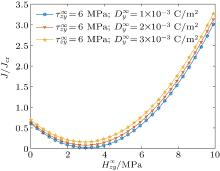Fig. 12.  Variations of J/Jcr with ${H}_{zy}^{\infty }$ for some given ${D}_{y}^{\infty }$ .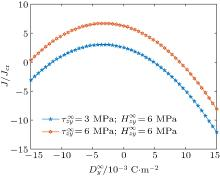Fig. 13.  Variations of J/Jcr with ${D}_{y}^{\infty }$ for some given ${\tau }_{zy}^{\infty }$ .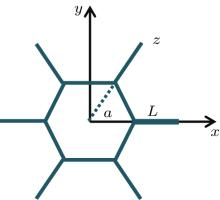Fig. A1.  z plane.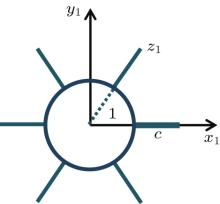Fig. A2.  z1 plane.Fig. A3.  z1 plane, $0\lt \theta \lt \displaystyle \frac{\pi }{3}$ .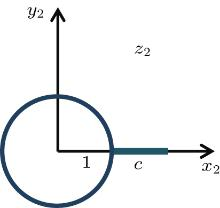Fig. A4.  z2 plane.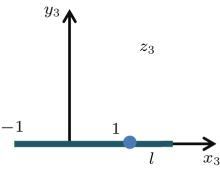Fig. A5.  z3 plane.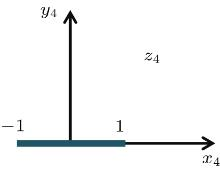Fig. A6.  z4 plane.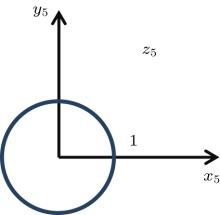Fig. A7.  z5 plane.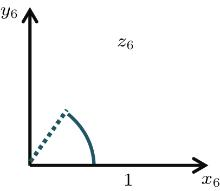Fig. A8.  z6 plane.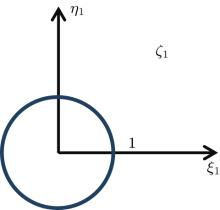Fig. A9.  ζ plane.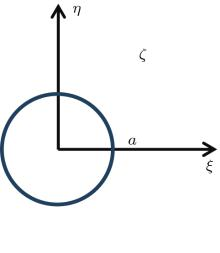Fig. A10.  ζ plane.
  Shechtman D, Blech I, Gratias D et al. 1984 Phys. Rev. Lett. 53 1951 DOI: 10.1103/PhysRevLett.53.1951  Liu G T, Fan T Y, Guo R P 2003 Mech. Res. Commun. 30 335 DOI: 10.1016/S0093-6413(03)00034-X  Liu G T, Fan T Y 2003 Sci. Chin. E 46 326 DOI: 10.1360/03ye9036  Liu G T, Fan T Y, Guo R P 2004 Int. J. Solids Struct. 41 3949 DOI: 10.1016/j.ijsolstr.2004.02.028  Guo J H, Liu G T 2008 Appl. Math. Mech-Engl. 29 485 DOI: 10.1007/s10483-008-0406-x  Guo J H, Liu G T 2007 Acta Math. Appl. Sin. 30 1066 in Chinese  Guo J H, Liu G T 2008 Chin. Phys. B 17 2610 DOI: 10.1088/1674-1056/17/7/044  Chen Z, Liu G, Guan L 2011 Chin. J. Solid Mech. 29 412 in Chinese  Zhong H Y, Liu G T 2015 Chin. J. Solid Mech. 36 179 in Chinese  Hou X L, Wang J X, Jia L G 2018 Chin. J. Appl. Mech. 35 484 in Chinese  Liu G T, Yang L Y 2017 Chin. Phys. B 26 094601 DOI: 10.1088/1674-1056/26/9/094601  Li L H, Liu G T 2012 Acta Phys. Sin. 61 086103 in Chinese DOI: 10.7498/aps.61.086103  Li L H, Liu G T 2009 Mod. Phys. Lett. B 23 3397 DOI: 10.1142/S0217984909021430  Wang X 2006 Mech. Res. Commun. 33 576 DOI: 10.1016/j.mechrescom.2005.02.022  Li X, Huo H S, Shi P P 2014 Chin. J. Solid Mech. 35 135 DOI: 10.19636/j.cnki.cjsm42-1250/o3.2014.02.004  Fu X L, Wang G F, Feng X Q 2010 Eng. Fract. Mech. 77 1048 DOI: 10.1016/j.engfracmech.2010.02.022  Kim C I, Schiavone P, Ru C Q 2011 Arch. Mech. 63 267  Gurtin M E, Murdoch A I 1975 Arch. Ration. Mech. Anal. 57 291 DOI: 10.1007/BF00261375  Gurtin M E, Murdoch A I 1978 Int. J. Solids Struct. 14 431 DOI: 10.1016/0020-7683(78)90008-2  Xiao J H, Xu Y L, Zhang F C 2018 Theor. Appl. Fract. Mech. 96 476 DOI: 10.1016/j.tafmec.2018.06.012  Guo J H, Li X F 2018 Acta Mech. 229 4251 DOI: 10.1007/s00707-018-2232-1  Liu Y Z, Guo J H, Zhang X Y 2019 ZAMM-Z Angew. Math. Mech. 99 e201900043 DOI: 10.1002/zamm.201900043  Guo J H, He L T, Liu Y Z, Li L H 2020 Theor. Appl. Fract. Mech. 107 102553 DOI: 10.1016/j.tafmec.2020.102553  Song J Z, Li H, He Y Z, Ou G B 2004 J. Harbin Eng. Univ. 25 558  Jia L G, Sun H D, Wang C G 2012 Eng. Mech. 29 147 in Chinese  Jiang L J, Liu G T 2017 Chin. Phys. B 26 044601 DOI: 10.1088/1674-1056/26/4/044601
  First-principles calculation of influences of La-doping on electronic structures of KNN lead-free ceramics Ting Wang(王挺), Yan-Chen Fan(樊晏辰), Jie Xing(邢洁), Ze Xu(徐泽), Geng Li(李庚), Ke Wang(王轲), Jia-Gang Wu(吴家刚), Jian-Guo Zhu(朱建国). Chin. Phys. B, 2020, 29(6): 067702.  First-principles study of polarization and piezoelectricity behavior in tetragonal PbTiO3-based superlattices Zhenye Zhu(朱振业). Chin. Phys. B, 2018, 27(2): 027701.  Multiferroic and enhanced microwave absorption induced by complex oxide interfaces Cuimei Cao(曹翠梅), Chunhui Dong(董春晖), Jinli Yao(幺金丽), Changjun Jiang(蒋长军). Chin. Phys. B, 2018, 27(1): 017503.  Piezoelectricity in K1-xNaxNbO3: First-principles calculation Li Qiang, Zhang Rui, Lv Tian-Quan, Zheng Li-Mei. Chin. Phys. B, 2015, 24(5): 053101.
 No Suggested Reading articles found!